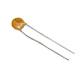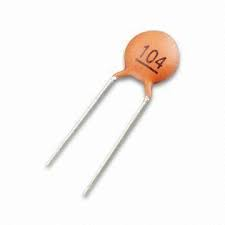Capacitors – Now we add letters to the mix

Adding letters to capacitor values isn't that complicated; it's just one more little detail to be aware of. The following sums up some basic letter codes for capacitor tolerances:
Some capacitors are defined by a three number code followed by a letter. This letter represents the tolerance of the capacitor, meaning how close the actual value of the capacitor can be expected to be to the indicated value of the capacitor. The tolerances are indicated as follows:
Read E as 0.5 percent. This is a duplication of a D code.
Read P as plus 100 percent to minus 0 percent.
Read Z as plus 80 percent to minus 20 percent.

Next week, we'll talk about more naming schemes, and wind up with a couple of links which illustrate just how (unnessarily?) complex this subject can become.

One of the many capacitor numbering schemes

This is a tiny ceramic capacitor.  You can only read the stuff printed on it under high magnificationLet's say that this is the same capacitor, but under high magnification. But what does '104' mean? Is it some kind of model number? Inventory number? Does it mean nothing to anyone but space aliens?Nope. It tells you it's capacitance, in picofrads. The first two digits indicate part of it's capacitance value. The third digit indicates the number of zeros which follow the first two digits.

Thus, it's capacitance is 10, followed by four zeros, i.e., 100,000 picofarads (100,000 pF)

You can also just drop the last three zeros and call it 100 nanofarads (100 nF). Both are the same value; they're just  written differently.

You can find out a bit more here.

And even more, in a straight-to-the-point video, here.

That strange stuff printed on capacitors

This begins a series of posts about stuff which is printed on the outside of capacitors. We'll deal with some of the schemes for indicating mF, uF, nf, and pF.

For our purposes:

1 mF is also known as
10 to the minus-3rd power of a Farad

1 uF is also known as
10 to the minus-6th power of a Farad

1 nF is also known as
10 to the minus-9th power of a Farad